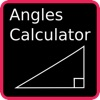Angles Calculator - By Essence Computing - Lumos Educational App Store4.51
Price -\$0.99
\$0.99

DESCRIPTION:

Do you need to learn how to determine the angles and lengths of a Right Angle Triangle? Need to clearly understand which of the sine, cosine and tangent ratios to use? Need to know how to determine the angle when you know two of the sides. If the answer is Yes to any of these questions then this App is for you. The Angles Calculator not only calculates the answers for you but it also tells you how it did it so you can understand how and when to apply the rules for right angle triangles. New this version: Now the area of the triangle is calculated for you. An explanation of how this was done is

OVERVIEW:

Angles Calculator - By Essence Computing is a free educational mobile app By Essence Computing.It helps students in grades 7 practice the following standards 7.G.5.

This page not only allows students and teachers download Angles Calculator - By Essence Computing but also find engaging Sample Questions, Videos, Pins, Worksheets, Books related to the following topics.

1. 7.G.5 : Use facts about supplementary, complementary, vertical, and adjacent angles in a multi-step problem to write and solve simple equations for an unknown angle in a figure. .

7

STANDARDS:

7.G.5

Developer: Essence Computing

Software Version: 1.7

Category: Education

RELATED APPSEdSearch WebSearch